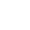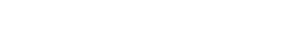## Accounting for overheads | Fixed Variable | Absorption rate

What constitutes part of inventory value?

Direct costs

• Direct material
• Direct labour
• Direct expenses

• Fixed Production (Depends on costing system used):

In Absorption costing charged to cost unit i,e Absorbed.

• Variable non-Production
1. Production (Depends on costing system used): In marginal costing treated as period cost and charged as an expense in the period in which they incur
2. Non-Production• Absorption costing requires a company to calculate a fixed overhead absorption rate, which is then used to measure the fixed overhead that relates to each unit of production.
• A company must undertake a series of steps in order to arrive at meaning full rates:
• Share the fixed production overhead to departments.
• Estimate fixed overhead absorption rate based on usage of resources in each department.

To reach an absorption rate, a company needs to find something which varies with production(this maybe total number of units or no. of hours worked) and divide the total fixed production overheads by that figure.• Overheads are allocated to cost centres. If a cost center is responsible for entire cost of an item, the entire cost is charged directly to the cost centre.
• Many items of indirect cost cannot be charged directly to a cost unit but can be charged directly to a cost centre, in that case charge items of expense in full to the cost centre.
• Fixed production may be allocated to:
• Production department/centre
• Service department/centre (that provide support to production department but not directly engaged in production).

Overhead costs that cannot be directly allocated to a cost centre must be share/apportioned between two or more cost centres.

Shared costs maybe divided between:

• selling and distribution cost centres,
• production cost centres,
• service cost centres.

Apportionment of production overheads costs goes in TWO stages:

• sharing general cost between production and services centres and; then
• sharing cost of service centres between production centres (secondary apportionment)After this has been done. The total overheads costs of each production centre should be:

• Cost allocated directly to the production centre, plus
• Share costs to production centres, plus
• Shared cost of service department apportioned to production centres.

The purpose of doing this is to calculate an absorption rate.• After production overheads have been allocated and apportioned to production departments and service departments, then the total for each service department is re-apportioned to the production department.
• The basis of re-apportionment of the overheads from each service department is some measure of how much its service is used by other departments.

This is called secondary apportionment.A service department might be used by production department and by another service department. In that case the secondary apportionment proceeds in TWO stages:

• the cost of service department used by other are apportioned first; and
• then the new total for the second service department is apportioned to production departments.

A situation may arise where both service department do work for the other service department as well as the production departments. In that case apportionment can be done in either of two methods below, each gives the same result:

• Repeated Distribution,
• Simultaneous Equation.• Taking each service department in turn, the overheads of that department are apportioned to all departments that use its service (i,e to the other service department as well as to the production department).
• This leaves the first service department with no overheads.
• The overheads of the second service department are then apportioned to all department that use its services (i,e to the other service department as well as to the production department).
• Repeat this process until all the overheads from service departments are apportioned to production departments leaving service departments with NIL balance.
• This method is to create two equations for the apportionment of service department overheads. These are simultaneous equations.
• The solution to the simultaneous equation can then be used to calculate the overhead apportionment to each production department.
• The following steps involves:

Step I

• establish two simultaneous equations, one for each service department. Each equation should state the total amount of overheads that will be apportioned from service department plus the proportion of the costs of the other service department that will be apportioned to it.

e.g:

X= F.O.H + %Y
Y= F.O.H + % X

Step II

• solve the equations to find value for X and Y.

Step III

• use the values of X and Y to establish total costs to apportion from service department to each production department.

*The purpose of doing this is to calculate an absorption rate.• Budgeted data is used rather than data about actual costs and output.
• Predetermined overhead absorption rates are calculated from;
• the budgeted volume/activity levels.

volume of activity in the period

An overhead absorption rate can be calculated for each production department separately or a single rate for all the production department in the factory (also known as Blanket Rate).• Actual overhead expenditure and actual production volume will almost be different from planned expenditure and production volume. This means that production overheads absorbed in product costs will be higher or lower than actual production overhead expenditure.
• This means there is some over absorption or under absorption.
 Over Absorption Under Absorption means expenses in comprehensive income are overstated.is added to profit in the cost accounting system.increases profit. is deducted from profit.reduces the reported profit.

*There is no adjustment to the value of closing inventory to allow over/under absorption in costing.